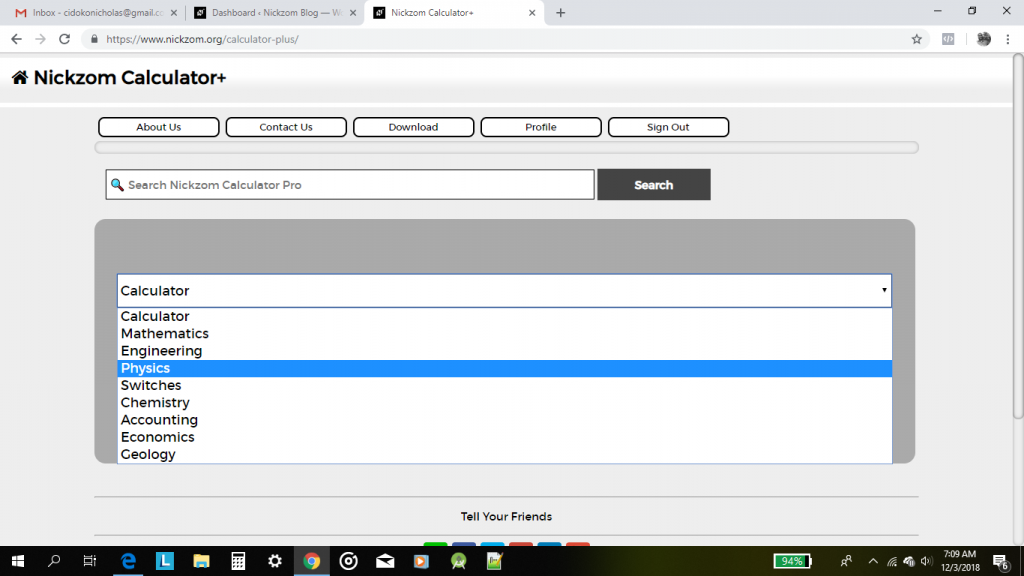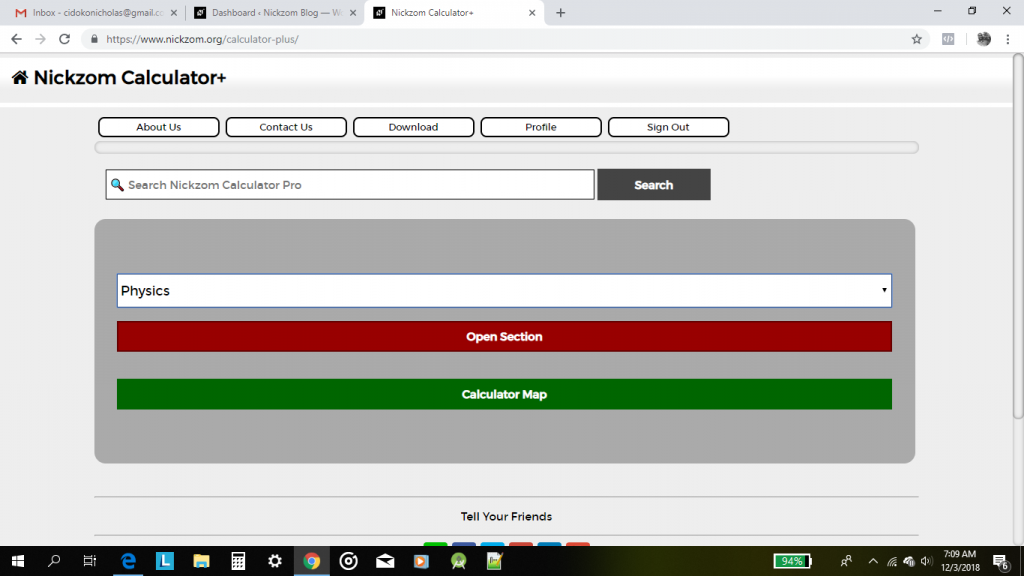## Nickzom Calculator Calculates Range and Maximum Range of a Projectile in Physics

To get the answer for the range of a projectile, there are three essential parameters to be given which are:

• Acceleration due to Gravity
• Initial Velocity
• Angle of Projection

Where as to get the answer for maximum range of a projectile requires two parameters to be given which are:

• Acceleration due to Gravity
• Initial Velocity

Let’s first, proceed to the home page of the app. Select Physics and click on Open SectionThen proceed to click on Projectile## Nickzom Calculator Calculates Maximum Height of a Projectile

According to SoftSchools.com,

A projectile is an object that is given an initial velocity, and is acted on by gravity. The maximum height of the object is the highest vertical position along its trajectory. The maximum height of the projectile depends on the initial velocity v0, the launch angle θ, and the acceleration due to gravity. The unit of maximum height is meters (m).

Nickzom Calculator calculates the maximum height of a projectile given the accurate values for the required parameters. The required parameters to get the answer to maximum height are:

• Acceleration due to Gravity
• Initial Velocity
• Angle of Projection

Let’s get started, first and foremost, proceed to the home page of the app, select Physics and click on Open Section.## Nickzom Calculator Calculates the Total Time of Flight and Time of Flight at Maximum Height in Projectile (Physics)

I am not hear to start explaining to you the meaning and application of time of flight. I believe you are here to know how the calculator encyclopedia calculates these parameters.

Nickzom Calculator requires three parameters to get the answer for time of flight and these parameters are:

• Acceleration due to Gravity
• Initial Velocity
• Angle of Projection

Let’s first get the workings and answer for time of flight at maximum height where the acceleration due to gravity is 10 m/s2, initial velocity is 20 m/s and angle of projection is 30°.

First and Foremost, Select Physics from the home page and click on Open SectionIn the Physics section, Click on Projectile